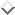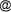## Lazare Tia, Sebastian Schmidtlein, Paul L. G. Vlek: Estimating and Upscaling Tree Density by Applying LAI-SEB Model to Optical Remotely Sensed Imagery within the Volta Basin (West Africa)

LAZARE TIA1, SEBASTIAN SCHMIDTLEIN2, PAUL L. G. VLEK1
1University of Bonn, Center for Development Research (ZEF), Germany
2University of Bonn, Geographical Institute, Germany

The most common certain way to determine tree density is to count the number of tree stems per unit area, given that most of ecological estimation outputs are subject to bias, and more, to uncertainty. The primary requirement for that accurate tree density estimate is field-based tree biometric measurements. But most of the time, that method of estimation appears to be expensive, difficult and time consuming for larger areas of investigation. Therefore, our alternative option consist of predicting tree density by means of optical remote sensing imagery through the development of an appropriate cross validated multivariate regression model - named LAI-SEB Model (Leaf Area Index - Surface Energy Balance Model) - which is applied to large regions within the Volta Basin. To achieve this goal, the Aster scene of Nov. 17, 2006, pixel size 30m is used. That satellite scene is processed according to standard methods such geometric correction using GCPs (Ground Control Points), image enhancement (histogram equalisation, atmospheric correction). Then, vegetation indexes and surfaces energy balance products such as SAVI, LAI, FPAR (Fraction of Absorbed Photosynthetically Active Radiation), Surface Albedo, Net Radiation, Absorbed Solar Radiation Flux, Sensible Heat Flux, and Latent Heat Flux are computed. For ground truthing, absolute tree density is obtained from tree biometric standard measurements based on systematic sampling method applied to quadrats of 30m by 30m. The mean tree density estimate in these field plots is 331.07 ( 3.76) tree stems ha-1; the standard deviation is 250.09; the minimum is 11.00 and the maximum is 1233.00 tree stems ha-1. The Partial Least Squares Regression (PLS"=R) method was used to predict tree density from the above mentioned image derivates. The prediction consist of, first, establishing a multivariate regression model between dependent and independent variables at site scale, and second, using the regression model on new X"=data set to predict new Y"=data at regional or Volta Basin scale.

Keywords: Tree density, LAI-SEB Model, multivariate regression, remote sensing

#### Footnotes

...PContact Address: Lazare Tia, University of Bonn, Center for Development Research (ZEF)Walter-Flex-Str. 3, 53113 Bonn, Germany, e-mail: ltiauni-bonn.de
Andreas Deininger, November 2007